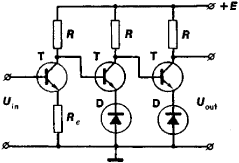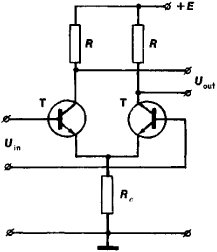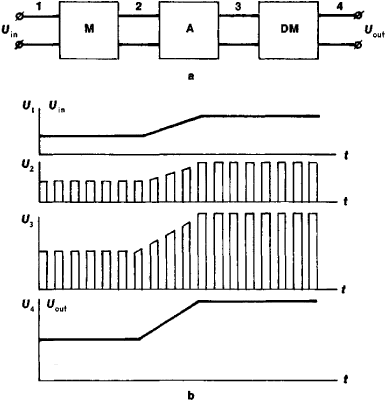# Direct-Current Amplifier

## direct-current amplifier

[də¦rekt ¦kə·rənt ′am·plə‚fī·ər]
(electronics)
An amplifier that is capable of amplifying dc voltages and slowly varying voltages.
McGraw-Hill Dictionary of Scientific & Technical Terms, 6E, Copyright © 2003 by The McGraw-Hill Companies, Inc.
The following article is from The Great Soviet Encyclopedia (1979). It might be outdated or ideologically biased.

## Direct-Current Amplifier

a transistor or vacuum-tube amplifier of electric signals that vary more or less slowly. It finds application in devices used in measurement technology and automation, where it is combined with a sensor, such as a photoelectric cell or a thermocouple. It is often used when small currents and charges are measured; a special type of DC amplifier tube has found acceptance as an electrometer. The DC amplifier is also used as an operational amplifier in analog computers. In the design and operation of DC amplifiers particular attention is paid to reducing the slow changes (drift) of the output voltage or current that occur in the absence of an input signal. Such changes are caused by a number of uncontrollable factors, such as aging of amplifier components, fluctuations of ambient temperature, and voltage fluctuations of the power supply.Figure 1. Schematic of a simple single-ended DC amplifier: (T) transistor, (R) load resistor, (Re) resistor in emitter circuit, (D) breakdown diode, (Uin) voltage at the input, (Uout) voltage at the output, (E) voltage of the power supply

Straight DC amplifiers are distinguished from DC amplifiers employing frequency conversion. The first type (Figures 1 and 2) is characterized by the absence of reactive elements such as capacitors or transformers in the coupling circuits; it was developed before the second type. In straight DC amplifiers the drift problem is solved by a direct reduction of drift in each of the amplifier stages, especially in the input stage. Differential stages are used for this purpose (Figure 2). In these stages the differential drift is kept at a minimum by a careful symmetrization of both arms of the circuit.Figure 2. Schematic of a simple differential (push-pull) stage of a DC amplifier: (T) transistor, (R) load resistor, (Re) coupling resistor for emitter circuits, (Uin) voltage at the input, (Uout) voltage at the output, (E) voltage of the power supply

In DC amplifiers with frequency conversion (Figure 3) the drift problem is solved by a conversion (modulation) of the slowly changing input signal by means of auxiliary oscillations—that is, by a conversion of the input signal into a signal whose frequency is that of the auxiliary oscillations and whose amplitude is proportional to the amplitude at the input. Subsequently, the converted signal is amplified by a drift-free amplifier with reactive coupling elements between stages. The signal is then rectified (demodulated) and thus again converted to a signal of the same shape as the input signal.

Present (1975) DC operational amplifiers have a gain as high as 106 and a passband from 0 to 100 megahertz. Over prolonged time periods (several tens of hours) and for a broad temperature range (–60° to + 100° C) their drift does not exceed a few tens of microvolts.

### REFERENCE

Erglis, K. E., and I. P. Stepanenko. Elektronnye usiliteli, 2nd ed. Moscow, 1964.

I. P. STEPANENKOFigure 3. A DC amplifier with frequency conversion: (a) schematic, (b) plots of signal voltage versus time at the points 1, 2, 3, and 4; (M) modulator; (A) drift-free amplifier; (DM) demodulator; (Uin) voltage at the input (Uout) voltage at the output; (U1). (U2), (U3). and (U4) voltages at the corresponding points of the amplifier; (t) time